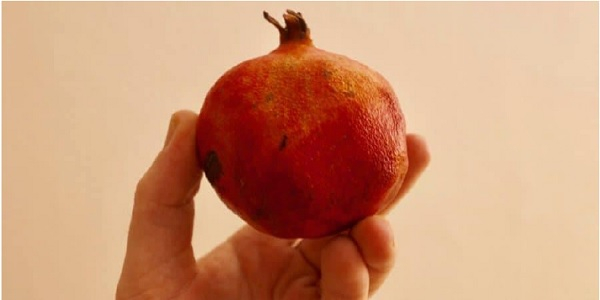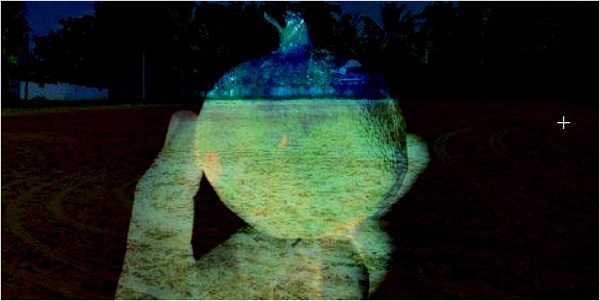# Arithmetic operations using OpenCV in Python

PythonServer Side ProgrammingProgramming

#### Beyond Basic Programming - Intermediate Python

Most Popular

36 Lectures 3 hours

#### Practical Machine Learning using Python

Best Seller

91 Lectures 23.5 hours

#### Practical Data Science using Python

22 Lectures 6 hours

In this tutorial, we are going to perform arithmetic operations on images using OpenCV in Python. We need to install the OpenCV module.

Run the following command to install the OpenCV module.

pip install opencv-python==4.1.1.26

If you run the above command, you will get the following successful message.

Collecting opencv-python==4.1.1.26
6221dd8d05119b9782d4c2b1d1482e22b5f5e/opencv_python-4.1.1.26-cp37-cp37m-win_amd64.w
hl (39.0MB)
Requirement already satisfied: numpy>=1.14.5 in c:\users\hafeezulkareem\anaconda3\l
ib\site-packages (from opencv-python==4.1.1.26) (1.16.2)
Installing collected packages: opencv-python
Successfully installed opencv-python-4.1.1.26

## Adding Two Images

We need two images for the addition. We have a method called cv2.add(image_one, image_two) to perform addition. It's very hand method. The sizes of the two images must be the same. Let's see the images.

Image OneImage twoLet's see the code.

### Example

# importing cv2 module
import cv2
# reading the images and storing in variables
# adding two images
result_image = cv2.add(image_one, image_two)
# displaying the final image
cv2.imshow('Final Image', result_image)
# deallocating the memory
if cv2.waitKey(0) & 0xff == 27:
cv2.destroyAllWindows()

### Output

Result Image## Subtraction

We have a method called cv2.substract(image_one, image_two) to perform subtraction on two images. We are going to use the same images as an addition. Let's see the code.

### Example

# importing cv2 module
import cv2
# reading the images and storing in variables
# substracting two images
result_image = cv2.subtract(image_one, image_two)
# displaying the final image
cv2.imshow('Final Image', result_image)
# deallocating the memory
if cv2.waitKey(0) & 0xff == 27:
cv2.destroyAllWindows()

### Output

Result Image## Conclusion

If you have any doubts regarding the tutorial, mention them in the comment section.